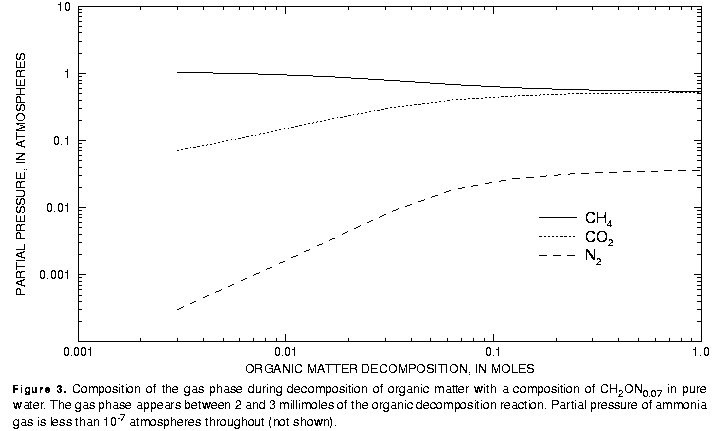[Next] [Previous] [Up] [Top]

EXAMPLES

## Example 7.-- Gas-Phase Calculations

This example demonstrates the capabilities of PHREEQC to model the appearance and evolution of a fixed-pressure multicomponent gas phase--a bubble. Gas-liquid reactions can be modeled in two ways with PHREEQC: a gas can react to maintain a fixed partial pressure using EQUILIBRIUM_PHASES keyword, or a fixed-total-pressure, multicomponent gas phase can be modeled using the GAS_PHASE keyword. Conceptually, the difference between the two approaches depends on the size of the gas reservoir. If the reservoir is essentially infinite, as in the atmosphere and unsaturated zone, then fixing the partial pressure of a gas is appropriate. If the reservoir is finite, as in gas bubbles in estuarine and lake sediments, then fixing the total pressure of the gas phase is appropriate. Here, the GAS_PHASE keyword is used to model the decomposition of organic matter in pure water, with the assumption that only carbon and nitrogen are released by the decomposition reaction. With no other electron acceptors available in pure water, the pertinent microbiological decomposition reaction is methanogenesis. The carbon and nitrogen released by organic decomposition are assumed to react to redox and gas-solution equilibrium. Aqueous carbon species are defined for two valence states, carbon(+4) and carbon(-4) (methane); no intermediate valence states of carbon are defined. Aqueous nitrogen may occur in the +5, +3, 0, and -3 valence states. The gases considered are carbon dioxide (CO2), methane (CH4), nitrogen (N2), and ammonia (NH3).

The initial water for this example is defined to be a ground water in equilibrium with calcite at a partial pressure of carbon dioxide of 10-1.5. Pure water is defined with the SOLUTION keyword by using defaults for all values (pH = 7, pe = 4, temperature = 25 C); calcite and carbon dioxide are defined with EQUILIBRIUM_PHASES; and SAVE is used to save the equilibrated solution (table 15). The organic decomposition reaction with a carbon to nitrogen ratio of approximately 15:1 is added irreversibly to this solution in increments ranging from 1 to 1000 mmol (REACTION keyword). A gas phase, which initially has no moles present, is allowed to form if the sum of the partial pressures exceeds 1.1 atm (GAS_PHASE keyword); only CO2, CH4, N2, and NH3 are allowed to occur in the gas phase. SELECTED_OUTPUT is used to print to a file (ex7.pun) the partial pressures and the number of moles in the gas phase of each gas at each step of the reaction.

### Table 15. Input data set for example 7

```TITLE Example 7.--Organic decomposition and bubble formation
SOLUTION 1
EQUILIBRIUM_PHASES 1
Calcite
CO2(g)  -1.5
SAVE solution 1
SELECTED_OUTPUT
-file ex7.pun
-si CO2(g) CH4(g) N2(g) NH3(g)
-gas CO2(g) CH4(g) N2(g) NH3(g)
END
USE solution 1
GAS_PHASE 1
-pressure       1.1
CO2(g)          0.0
CH4(g)          0.0
N2(g)           0.0
NH3(g)          0.0
REACTION 1
(CH2O)N0.07     1.0
1. 2. 3. 4. 8. 16. 32 64. 125. 250. 500. 1000. mmol
END
```
The gas phase appears between 2 and 3 mmol of reaction have been added (fig. 3). Initially the gas is more than 90 percent CH4 and less than 10 percent CO2, with only minor amounts of N2 and NH3 (NH3 partial pressures were less than 10-7 atm throughout the reaction calculation). The volume of gas produced ranges from less than 1 mL at 3 mmol of reaction to more than 20 L after 1 mol of reaction. After 1 mol of reaction is added, nearly all of the carbon and nitrogen is in the gas phase.Table 15. Input data set for example 7

User's Guide to PHREEQC - 07 MAY 96
[Next] [Previous] [Up] [Top]